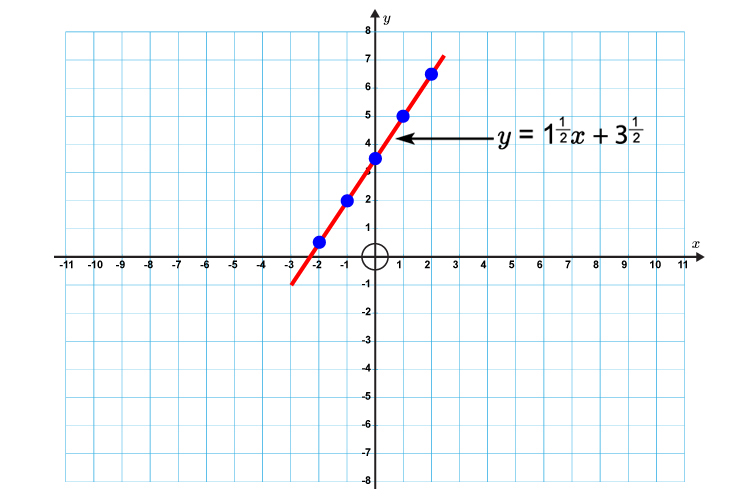# Plotting lines on graph paper

Example 1

Plot the graph of  y = x

This actually means y=mx+c

y=1x+zero

## Graph of y=x

First lets calculate some points on the graph.

x=-2      then   y=-2

x=-1      then   y=-1

x=0           then    y=0

x=1           then   y=1

x=2           then   y=2

Now draw a graph and mark on the above points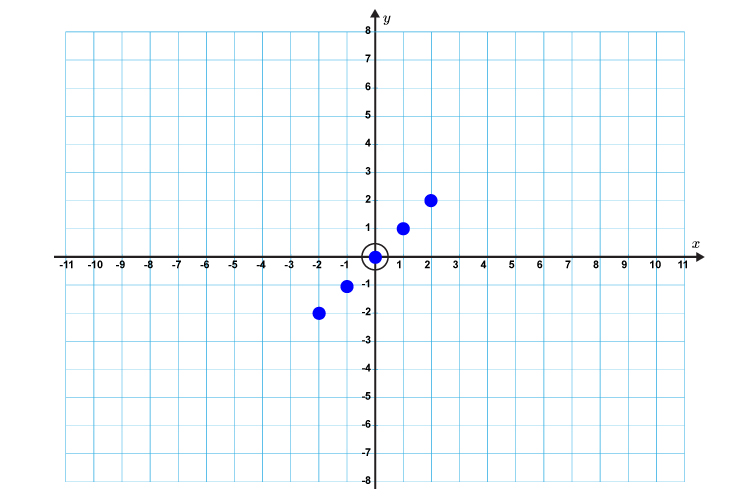Now draw a straight line through these points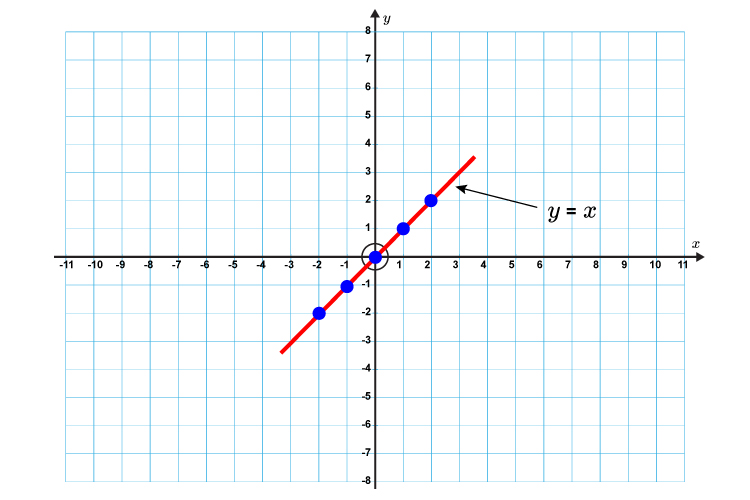Example 2

Plot the graph of  y+x=5

Always put the formula in the form  y=mx+c

y=-1x+5

First lets calculate some points on the graph.

x=-2    then y=-1(-2)+5=7

x=-1    then y=-1(-1)+5=6

x=0         then y=-1(0)+5=5

x=1         then y=-1(1)+5=4

x=2        then y=-1(2)+5=3

Now draw a graph and mark on the above points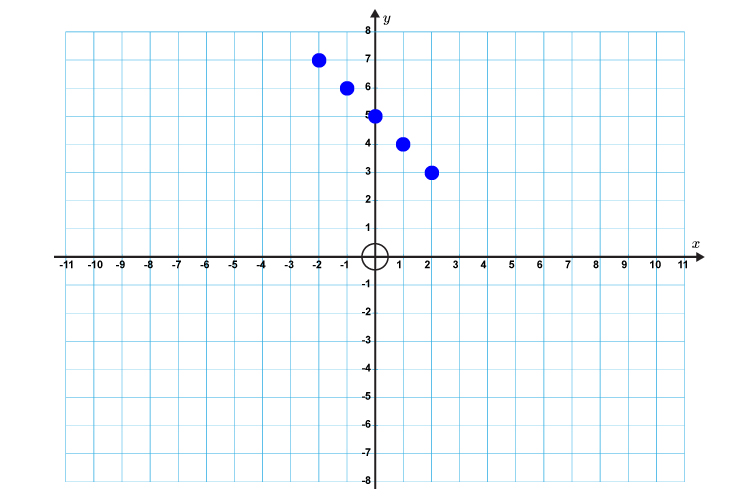Now draw a straight line through these points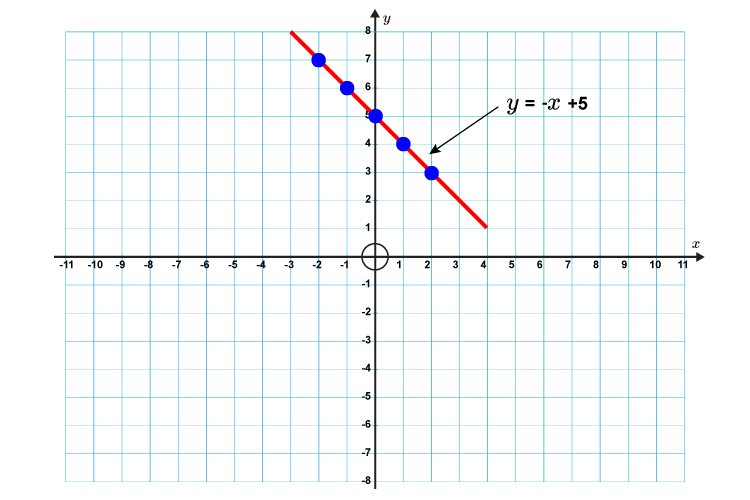Example 3

Plot the graph of   2y -3x=7

Always put the formula in the form    y=mx+c

2y=3x+7

y=3/2x+7/2

y=1\1/2x+3\1/2

First lets calculate some points on the graph.

x=-2   then y=1.5(-2)+3.5=0.5

x=-1   then y=1.5(-1)+3.5=2

x=0         then y=1.5(0)+3.5=3.5

x=1         then y=1.5(1)+3.5=5

x=2        then y=1.5(2)+3.5=6.5

Now draw a graph and mark on the above points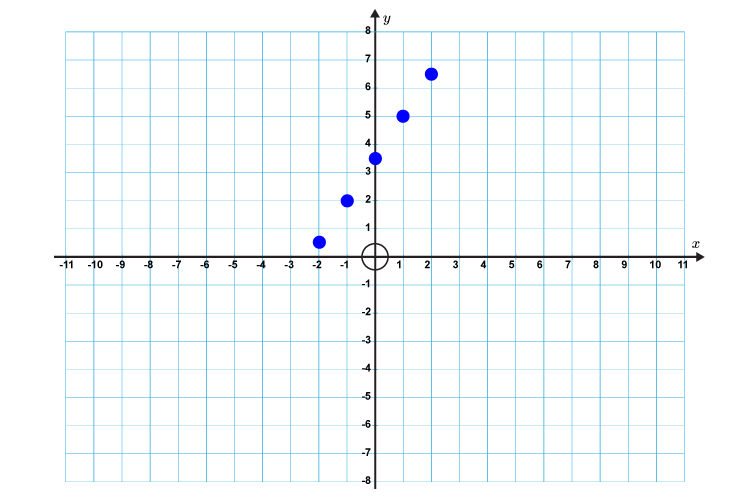Now draw a straight line through these points